# Redistricting with Flip MCMC

library(redist)
library(ggplot2)
library(dplyr)
library(patchwork)
# set seed for reproducibility
set.seed(1)

The redist package is designed to allow for replicable redistricting simulations. This vignette covers the Flip Markov Chain Monte Carlo method discussed in: Automated Redistricting Simulation Using Markov Chain Monte Carlo.

# The Flip MCMC Algorithm

data(fl25)
data(fl25_enum)
plan <- fl25_enum$plans[, 7241] adj <- redist.adjacency(fl25) set.seed(1) sims <- redist.flip(adj = adj, init_plan = plan, total_pop = fl25$pop,
nsims = 6, pop_tol = 0.10,
constraint = 'compact', constraintweights = 0.02,
compactness_metric = 'edges-removed')
#>
#> ====================
#> redist.flip(): Automated Redistricting Simulation Using
#>          Markov Chain Monte Carlo
#>
#> Preprocessing data.
#>
#> Starting swMH().
#> 10 percent done.
#> Metropolis acceptance ratio: inf
#>
#> 20 percent done.
#> Metropolis acceptance ratio: 2
#>
#> 30 percent done.
#> Metropolis acceptance ratio: 1.5
#>
#> 40 percent done.
#> Metropolis acceptance ratio: 1.33333
#>
#> 50 percent done.
#> Metropolis acceptance ratio: 1.25
#>
#> 60 percent done.
#> Metropolis acceptance ratio: 1.2

The flip algorithm is one of the more straightforward redistricting algorithms. Beginning with an initial partition of a graph, it proposes flipping a node from one partition to an adjacent partition. By checking that the proposed flip meets basic constraints, such as keeping partitions contiguous and staying within a certain population parity, it ensures that all proposed new partitions are also valid partitions. The implementation within redist is a bit more advanced that this, as it allows for multiple flips and rejecting valid partitions based on a Metropolis Hastings algorithm. The following walks through the basics of this algorithm to provide an introduction to using flip correctly and efficiently.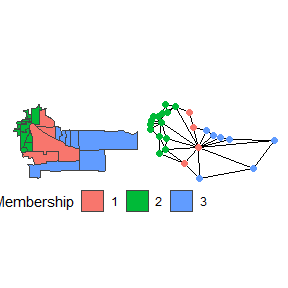Suppose we are redistricting this small map above on the left. To use the flip algorithm, we need to consider the adjacency graph that underlies this map, which is above on the right. Each of the 25 precincts on the left are displayed as a node on the right, connected if they are contiguous on the map. If we use the above district as an initial plan, we can then run flip for a few steps.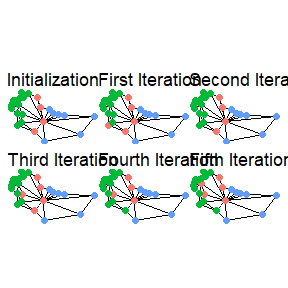While this map is extremely small, the five iterations give a basic idea of what is going on behind the scenes. At each iteration, it searches the boundary for possible swaps, selects one, and then accepts or rejects the proposals. With very weak constraints, like those used to create the above example, almost every swap is accepted. Even then, though, it doesn’t guarantee that some iterations won’t repeat other plans sampled. In fact, in the above, the second iteration is the same plan as the initialization.

This possibility is very important for ensuring that the sampled plans are representative of the desired target distribution, which is controlled by the constraints chosen. The possible constraints are discussed below, as is information on setting up simulations and some advice on ensuring that your simulations are efficient.

# Strengths of Flip

Flip is incredibly powerful for local exploration. If you can make large changes to a summary statistic of interest without making large changes to the map itself, this may tell an important story of what went into making the map.

Flip is one of the easiest to understand algorithms and has theoretical guarantees behind it. This can make it especially useful when the audience of interest does not have an advanced background in mathematics or statistics.

Flip has the power to make less compact maps than many other algorithms. This can be especially powerful when a blind allegiance to compactness makes otherwise viable plans appear to be outliers.

Our implementation of flip has many more Gibbs constraints than our other implementations. This can allow you to consider different forms of partisan and countysplit constraints among others.

However, with these strengths do come weaknesses. Like most Markov Chain Monte Carlo methods, convergence can’t be shown, it can only be suggested. Diagnostics, like those in the section on diagnostic plots, can help ensure that convergence is likely, but can never show that it has indeed happened. Additionally, flip makes relatively small moves per iteration, so many more iterations are needed to move around the space. If your map is particularly large, you may require several hundred iterations to make the map substantively different, which leads to thinning the chain, which is dropping many sequential iterations. However, thinning doesn’t make the algorithm more efficient, so you still need to work through those plans, which comes with a time cost.

# Initializing Flip

One of the keys to ensuring good performance is the choice of initialization. In some cases, a starting point may be obvious, such as when you want to explore the local area around an existing map. If that’s the use case, then it is straightforward to use that plan as the starting point. However, if the goal is to understand the larger space of possibilities, then starting from just one map can be misleading. Why? Since constraint tuning is not a perfect science, you could be setting the constraints too strong and, if that map is very good on some dimension, the flip algorithm may have difficulty getting away from that point without a very large number of iterations.

Our implementation defaults to using the Sequential Monte Carlo (SMC)algorithm via redist.smc()/redist_smc() to create an initial partition of the districts, if no district is provided.

While the implementations of Random Seed and Grow (RSG) and Compact Random Seed and Grow (CRSG) via redist.rsg() and redist.crsg do not sample from a defined target distribution, they can serve as useful initializations for flip as they help provide a more diverse set of starting states. SMC is often faster and provides more theoretical guarantees, but tends to sample very compact districts, even when decreasing the compactness constraint. As such, when trying to decide if chains have likely converged or not, it can be misleading to only check chains that start from very compact states.

# Redistricting with Flip MCMC

With the basics of what the flip algorithm is doing down, we can proceed into how to use the algorithm.

There are currently two ways to use flip within the redist package: via the standard interface (redist.flip) or via the tidy interface (redist_flip). This section covers the basics using the former. See the section on the tidy interface below and the tidy vignette for more information.

To begin running the MCMC algorithm, we have to provide some basic information, typically beginning with a shapefile. The below loads an Iowa dataset included within the redist package and plots the actual congressional districts from 2012-2021. (Iowa is a favorite choice for redistricting simulation examples, as it requires keeping counties together in plans which allows us to use the counties as the unit for redistricting, rather than thousands of precincts.)

data(iowa)
redist.plot.map(iowa, plan = cd_2010)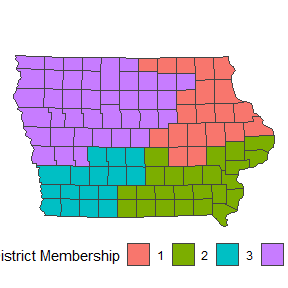From there, we need to build an adjacency graph which identifies which counties are touching which other counties on a map. If you have an existing plan, it’s generally advised to supply this to the optional plan argument to ensure that the existing plan is a valid, connected plan. If you get a warning, the geomander R package can help solve potential issues.

adj <- redist.adjacency(shp = iowa, plan = iowa$cd_2010) In addition, we need population for each unit. We’ve included iowa$pop as the total population as of the 2010 Census. From there, we have the basic information that we need to run our first simulation. The below indicates that we are simulating 1000 plans (with nsims) for the state of Iowa that have at most a population parity deviation of 0.05 (with pop_tol).

sims <- redist.flip(adj = adj, total_pop = iowa$pop, init_plan = iowa$cd_2010,
nsims = 100, pop_tol = 0.05)
#>
#> ====================
#> redist.flip(): Automated Redistricting Simulation Using
#>          Markov Chain Monte Carlo
#>
#> Preprocessing data.
#>
#> Starting swMH().
#> 10 percent done.
#> Metropolis acceptance ratio: 1.11111
#>
#> 20 percent done.
#> Metropolis acceptance ratio: 0.947368
#>
#> 30 percent done.
#> Metropolis acceptance ratio: 0.965517
#>
#> 40 percent done.
#> Metropolis acceptance ratio: 0.974359
#>
#> 50 percent done.
#> Metropolis acceptance ratio: 0.979592
#>
#> 60 percent done.
#> Metropolis acceptance ratio: 0.949153
#>
#> 70 percent done.
#> Metropolis acceptance ratio: 0.956522
#>
#> 80 percent done.
#> Metropolis acceptance ratio: 0.936709
#>
#> 90 percent done.
#> Metropolis acceptance ratio: 0.921348
#>
#> 100 percent done.
#> Metropolis acceptance ratio: 0.929293

The printed output can be silenced by setting verbose = FALSE, however it displays very important information. First, it displays when preprocessing begins and when the algorithm actually starts. Each 10% of the way through the flip algorithm, it outputs the current estimated Metropolis acceptance. Here, we’ve specified no Gibbs constraints, so the acceptance will always be near 100%.

The output is an object of class redist.

class(sims)
#>  "redist"

The sims object includes various pieces of information that were tracked while simulating, but we focus on sims$plans, which is a matrix that contains the plans. dim(sims$plans)
#>   99 100

Checking the dimensions shows that each plan is saved as a column, where each row is a precinct. From this, we can extract a single plan as we would from a normal matrix, like below, where we plot the final simulated plan.

redist.plot.map(shp = iowa, plan = sims$plans[, 100])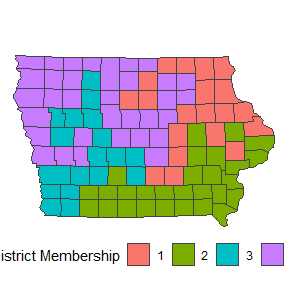Now, this plan is incredibly non-compact, which can be an issue. However, we should expect this type of outcome, as we didn’t include a compactness constraint while simulating. Thus, the only things checked were contiguity and that no plan would be outside of the pop_tol set above. Since there are many more non-compact plans than compact plans in the space of all redistricting plans, we end up with highly non-compact districts. We can fix this by specifying a constraint, as below: sims_comp <- redist.flip(adj = adj, total_pop = iowa$pop, init_plan = iowa$cd_2010, nsims = 100, pop_tol = 0.05, constraint = 'compact', constraintweights = 0.4, compactness_metric = 'edges-removed') #> #> ==================== #> redist.flip(): Automated Redistricting Simulation Using #> Markov Chain Monte Carlo #> #> Preprocessing data. #> #> Starting swMH(). #> 10 percent done. #> Metropolis acceptance ratio: 0.333333 #> #> 20 percent done. #> Metropolis acceptance ratio: 0.315789 #> #> 30 percent done. #> Metropolis acceptance ratio: 0.310345 #> #> 40 percent done. #> Metropolis acceptance ratio: 0.410256 #> #> 50 percent done. #> Metropolis acceptance ratio: 0.530612 #> #> 60 percent done. #> Metropolis acceptance ratio: 0.542373 #> #> 70 percent done. #> Metropolis acceptance ratio: 0.565217 #> #> 80 percent done. #> Metropolis acceptance ratio: 0.56962 #> #> 90 percent done. #> Metropolis acceptance ratio: 0.573034 #> #> 100 percent done. #> Metropolis acceptance ratio: 0.545455 The first arguments as the same, but this adds three key arguments. First, setting constraint to any combination of the nine implemented constraints allows us to specify the target distribution. Setting constraintweights = 0.4 means that we want to put a relatively weak weight on the compactness, though a weak constraint still does a lot of work. There are four compact constraints implemented currently. The recommended is to use edges-removed because it can be calculated very quickly. If we plot the final map sampled from the above code, we can see that it is far more compact. redist.plot.map(shp = iowa, plan = sims_comp$plans[, 100])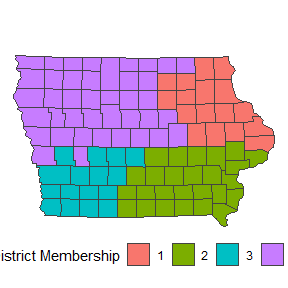# Using Multiple Chains

When running larger redistricting analyses, one important step is to run multiple chains of the MCMC algorithm. This will also allow us to diagnose convergence better, using the Gelman-Rubin plot, as seen in the section on Diagnostic Plots.

On Windows and in smaller capacities, it is useful to run the algorithm within an lapply loop. First, we set up the seed for replicability and decide on the number of chains and simulations.

set.seed(1)
nchains <- 4
nsims <- 100

Here, we opt to initialize using the RSG algorithm, though the default is to use the SMC algorithm. When we want to initialize without providing an initial partition, we need to specify the number of districts, ndists.

flip_chains <- lapply(1:nchains, function(x){
redist.flip(adj = adj,  total_pop = iowa$pop, pop_tol = 0.05, nsims = nsims, init_plan = 'rsg', ndists = 4, constraint = 'compact', constraintweights = 0.4, compactness_metric = 'edges-removed', verbose = FALSE) }) In Unix-based systems, this can be run considerably faster by running this in parallel. mcmc_chains <- parallel::mclapply(1:nchains, function(x){ redist.flip(adjobj = adjlist, popvec = fl25$pop,
nsims = nsims, ndists = 3)
}, mc.set.seed = 1, mc.cores = parallel::detectCores())

# Tidy Flip MCMC using redist_flip()

The new, tidy interface to functions with redist introduces a pair of key objects, redist_map and redist_plans. The Get Started page goes into depth about these, but this shows the basics of how to work with the flip algorithm within the newer interface.

As in the standard interface, we need a data set to work with. This example will also follow with using the included Iowa data.

data(iowa)

Rather than building the adjacency graph manually, here we can set this up using redist_map which will build it an add it as a column.

iowa_map <- redist_map(iowa, existing_plan = cd_2010, pop_tol=0.01)

We set a population tolerance of 1%. While this is generally a good population parity tolerance for most simulations, be careful when using the default within flip. If your starting partition sits outside of that population deviation, flip may take a very, very long time to find a valid partition to flip.

Now, we can pass the redist_map object to redist_flip to begin simulating.

tidy_sims <- iowa_map %>% redist_flip(nsims = 100)
#>
#> ====================
#> redist.flip(): Automated Redistricting Simulation Using
#>          Markov Chain Monte Carlo
#>
#> Preprocessing data.
#>
#> Starting swMH().
#> 10 percent done.
#> Metropolis acceptance ratio: 0.777778
#>
#> 20 percent done.
#> Metropolis acceptance ratio: 0.736842
#>
#> 30 percent done.
#> Metropolis acceptance ratio: 0.655172
#>
#> 40 percent done.
#> Metropolis acceptance ratio: 0.615385
#>
#> 50 percent done.
#> Metropolis acceptance ratio: 0.673469
#>
#> 60 percent done.
#> Metropolis acceptance ratio: 0.661017
#>
#> 70 percent done.
#> Metropolis acceptance ratio: 0.637681
#>
#> 80 percent done.
#> Metropolis acceptance ratio: 0.632911
#>
#> 90 percent done.
#> Metropolis acceptance ratio: 0.651685
#>
#> 100 percent done.
#> Metropolis acceptance ratio: 0.626263

This highlights a key difference between the redist_flip and redist.flip implementations: redist_flip appears to have a much lower acceptance rate, even though we didn’t explicitly set any constraints. Why? redist_flip’s constraint includes a relatively weak compactness constraint by default because simulating compact maps is far more efficient and completely non-compact maps are not super useful for most purposes.

You can override this using the flip_constraints_helper() function to set up a blank set of constraints.

cons <- flip_constraints_helper(iowa_map, constraint = NULL)

Then, you can pass this to redist_flip.

tidy_sims_no_comp <- iowa_map %>% redist_flip(nsims = 100, constraints = cons)
#>
#> ====================
#> redist.flip(): Automated Redistricting Simulation Using
#>          Markov Chain Monte Carlo
#>
#> Preprocessing data.
#>
#> Starting swMH().
#> 10 percent done.
#> Metropolis acceptance ratio: 1.11111
#>
#> 20 percent done.
#> Metropolis acceptance ratio: 1.05263
#>
#> 30 percent done.
#> Metropolis acceptance ratio: 0.965517
#>
#> 40 percent done.
#> Metropolis acceptance ratio: 0.948718
#>
#> 50 percent done.
#> Metropolis acceptance ratio: 0.959184
#>
#> 60 percent done.
#> Metropolis acceptance ratio: 0.949153
#>
#> 70 percent done.
#> Metropolis acceptance ratio: 0.942029
#>
#> 80 percent done.
#> Metropolis acceptance ratio: 0.949367
#>
#> 90 percent done.
#> Metropolis acceptance ratio: 0.955056
#>
#> 100 percent done.
#> Metropolis acceptance ratio: 0.939394

This increases the acceptance ratio to near 100% again. The function flip_constraints_helper() is useful for setting up any constraints to be used with redist_flip. For example, if we want to set up a compactness constraint and a similarity constraint (which is the flip status quo constraint), we can do so as follows:

cons <- flip_constraints_helper(map = iowa_map, constraint = c('compact', 'similarity'),
constraintweight = c(0.6, 1), init_plan = cd_2010)

From there, we can again pass this to redist_flip

tidy_sims_sq_comp <- iowa_map %>% redist_flip(nsims = 100, constraints = cons)
#>
#> ====================
#> redist.flip(): Automated Redistricting Simulation Using
#>          Markov Chain Monte Carlo
#>
#> Preprocessing data.
#>
#> Starting swMH().
#> 10 percent done.
#> Metropolis acceptance ratio: 0.777778
#>
#> 20 percent done.
#> Metropolis acceptance ratio: 0.631579
#>
#> 30 percent done.
#> Metropolis acceptance ratio: 0.551724
#>
#> 40 percent done.
#> Metropolis acceptance ratio: 0.641026
#>
#> 50 percent done.
#> Metropolis acceptance ratio: 0.55102
#>
#> 60 percent done.
#> Metropolis acceptance ratio: 0.610169
#>
#> 70 percent done.
#> Metropolis acceptance ratio: 0.594203
#>
#> 80 percent done.
#> Metropolis acceptance ratio: 0.556962
#>
#> 90 percent done.
#> Metropolis acceptance ratio: 0.539326
#>
#> 100 percent done.
#> Metropolis acceptance ratio: 0.545455

The output from redist_flip differs a bit from the output of redist.flip. It outputs a redist_plans object.

class(tidy_sims)
#>  "redist_plans" "tbl_df"       "tbl"          "data.frame"

To extract the plans, use get_plans_matrix().

plans <- get_plans_matrix(tidy_sims)

Alternatively, you can directly use functions on the redist_plans object. For example, if we want to measure the competitiveness of each plan:

tidy_sims <- tidy_sims %>%
mutate(competitiveness = competitiveness(map = iowa_map, rvote = rep_08, dvote = dem_08))
tidy_sims %>%
ggplot(aes(x = competitiveness)) +
geom_density() +
theme_bw()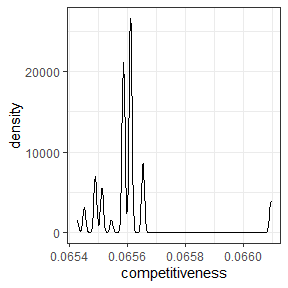For more information on using redist_plans objects, see the Get Started page.

# Diagnostic Plots

When using the MCMC algorithms, there are various useful diagnostic plots. The redist.diagplot function creates familiar plots by converting numeric entries into mcmc objects to use with coda.

We use the dissimilarity index in Massey and Denton 1988 as a summary statistic for the following examples. This can be computed with segregation_index or redist.segcalc. In this case, we create a Republican dissimilarity index. We can work with two examples, the first is a single vector of the segregation index, while the second is a list of vectors, with one vector for each chain.

seg <- redist.segcalc(plans = get_plans_matrix(tidy_sims),
group_pop = iowa_map$rep_08, total_pop = iowa_map$pop)

The first three plots only need a single index.

• Autocorrelation Plot
redist.diagplot(seg, plot = "autocorr")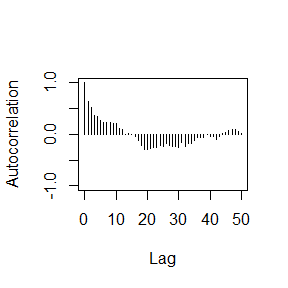• Density Plot
redist.diagplot(seg, plot = "densplot")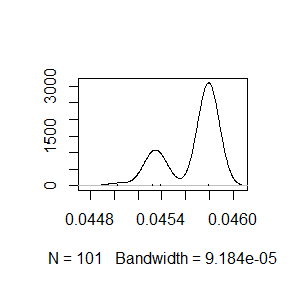• Mean Plot
redist.diagplot(seg, plot = "mean")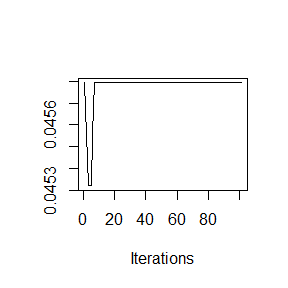As examples for the next two plots, we can use the example above which ran 4 chains. This is the same index, but computed for each chain.

seg_chains <- lapply(1:nchains,
function(i){redist.segcalc(plans = flip_chains[[i]],
group_pop = iowa_map$rep_08, total_pop = iowa_map$pop)})
• Trace Plot
redist.diagplot(sumstat = seg_chains, plot = "trace")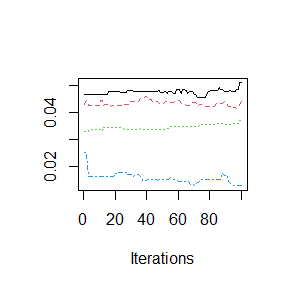• Gelman Rubin Plot
redist.diagplot(sumstat = seg_chains, plot = 'gelmanrubin')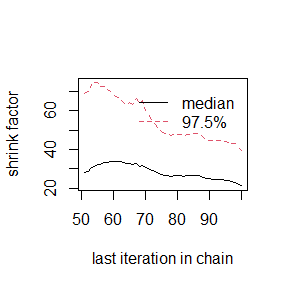# Tuning Flip Constraints

When using the flip algorithm, the most important and difficult step is setting the right constraint weights. While there may be some general pieces of advice for doing so, no advice can replace working with your data. The bottom line is that every data set is a bit different. What works for one state’s redistricting process, with the data specific to that state at that time may not transfer to another state or municipality or school district. The general process of finding what works might be very similar, but getting the right set of constraint weights and other parameters will vary immensely. Even starting from a different plan within the same time and place can change the weights that perform best. Like most things, the key to tuning flip is patience. Going for a full scale simulation without testing some parameter configurations is likely an inefficient use of time and computing power.

The following highlights some advice on how to tune flip to make it work for your particular redistricting problem. For the advice, we’ll use the following example:

data(iowa)
iowa_map <- redist_map(iowa, existing_plan = cd_2010, pop_tol = 0.02, total_pop = pop)

cons <- flip_constraints_helper(map = iowa_map, constraint = c('compact', 'population'),
constraintweight = c(0.5, 100))
sims <- redist_flip(map = iowa_map,  nsims = 100)
#>
#> ====================
#> redist.flip(): Automated Redistricting Simulation Using
#>          Markov Chain Monte Carlo
#>
#> Preprocessing data.
#>
#> Starting swMH().
#> 10 percent done.
#> Metropolis acceptance ratio: 0.444444
#>
#> 20 percent done.
#> Metropolis acceptance ratio: 0.368421
#>
#> 30 percent done.
#> Metropolis acceptance ratio: 0.448276
#>
#> 40 percent done.
#> Metropolis acceptance ratio: 0.410256
#>
#> 50 percent done.
#> Metropolis acceptance ratio: 0.367347
#>
#> 60 percent done.
#> Metropolis acceptance ratio: 0.322034
#>
#> 70 percent done.
#> Metropolis acceptance ratio: 0.333333
#>
#> 80 percent done.
#> Metropolis acceptance ratio: 0.329114
#>
#> 90 percent done.
#> Metropolis acceptance ratio: 0.337079
#>
#> 100 percent done.
#> Metropolis acceptance ratio: 0.323232

## Acceptance Ratios

One of the first things to check when working with flip is the Metropolis Hastings ratio. It is printed to the console when verbose = TRUE. If you have silenced printing or warnings, the output saves the Metropolis Hastings decisions. You can check the acceptance ratio in a redist_plans object with

mean(sims$mhdecisions, na.rm = TRUE) #>  0.32 Reference plans included in the object will not have an mhdecision, so you can remove them with na.rm = TRUE. The goal is to generally have the Metropolis Hastings ratio lie between 20% and 40%. If simulating with only a single parameter, the goal is generally to be near 40%, while with many parameters, you likely want to be near 20%. If over the course of many simulations you find yourself just above or just below, that probably isn’t a problem if the simulations are in the right probability space. ## lambda and eprob lambda and eprob both control the amount of movement within flip. They can be very powerful things to increase. lambda defaults to 0, while eprob defaults to 0.05. Each of these parameters leads to fairly small movements between sequential iterations of the algorithm. sims_new <- redist_flip(map = iowa_map, nsims = 100, pop_tol = 0.02, constraints = cons, eprob = 0.10, lambda = 2, verbose = FALSE) mean(sims_new$mhdecisions, na.rm = TRUE)
#>  0.12

In this example, we’ve increased each of these. lambda = 2, up from its default of 0, while eprob = 0.10, up from its default of 0.05. What’s going on here can characterized fairly well by the Hamming distance between sequential runs.

dists <- redist.distances(plans = get_plans_matrix(sims))$Hamming dists_new <- redist.distances(plans = get_plans_matrix(sims_new))$Hamming
for(i in 1:100){
adj_dists[i] <- dists[i, i + 1]
adj_dists_new[i] <- dists_new[i, i + 1]
}
lambda/eprob = c(rep('0/0.05', 100), rep('2/0.10', 100))) %>%
ggplot() +
geom_density(aes(x = Hamming, color = lambda/eprob)) +
theme_bw()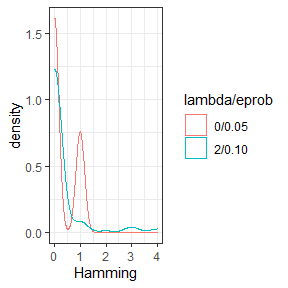lambda controls the number of components swapped between each iterations, while eprob controls the size of the swapped partitions. Increasing each of this values can be important for increasing the amount of movement between outputted plans. These can be adjusted automatically using adapt_lambda and adapt_lambda when starting a simulation, though adjusting them manually to fit your problem is better practice, as it leads to more control over the process.

## Adjusting pop_tol

Sometimes a starting map sits in a neighborhood of maps that isn’t very conducive to using it as a starting point. This is most often characterized by running a single iteration that runs (seemingly) forever. A typical fix for this is to weaken the population tolerance and use a Gibbs constraint to pull the simulations back into the target range. I’ve done this for the tuning example, even though it’s unnecessary.

After simulating, if there is a hard constraint to consider, we can check the parities:

sims <- sims %>% mutate(par = plan_parity(map = iowa_map))

And then we can subset to the correct space.

sims <- sims %>% filter(par <= 0.01)

With the right set of parameters, this will lead to a reasonable set of simulations. In this case, we end up with about 10% of the simulations when using a soft constraint, which is not uncommon. In general, you want to aim for as low as a hard population parity as possible, while using a strong weight on the Gibbs population when the hard constraint is above what’s necessary. This helps maximize the efficiency of your simulations, while allowing for additional movement between neighborhoods of valid plans.

## Balancing Multiple Constraints

More often than not, there are multiple constraints that are important to a redistricting problem. There are two general paths to success when working with more than one or two constraints.

First, you might want to add one at a time, generally starting with the compactness constraint. If flip doesn’t consider compactness at all, it has an unfortunate behavior of creating incredibly non-compact maps. However, with even a very weak compactness constraint, it performs very well in avoiding those maps that are so non-compact that they aren’t worthy of consideration. Then you can add the next constraints once at a time, weakening them a bit each time you add a new constraint. As above, you want to make sure that your acceptance rate is between 20% and 40%. If it’s too low, you won’t get sufficient movement around the probability space and if it’s too high, you likely aren’t characterizing the probability space you want to characterize.

The other way to tune is to run a simulation with a kitchen sink type set up.

cons <- flip_constraints_helper(map = iowa_map,
constraint = c('compact', 'population', 'partisan', 'countsplit'),
constraintweight = c(0.25, 50, 10, 10),
counties = name,
rvote = iowa$rep_08, dvote = iowa$dem_08)

Then we can run this for a relatively small number of iterations.

sims <- redist_flip(iowa_map, 100, constraints = cons)
#>
#> ====================
#> redist.flip(): Automated Redistricting Simulation Using
#>          Markov Chain Monte Carlo
#>
#> Preprocessing data.
#>
#> Starting swMH().
#> 10 percent done.
#> Metropolis acceptance ratio: 1
#>
#> 20 percent done.
#> Metropolis acceptance ratio: 0.947368
#>
#> 30 percent done.
#> Metropolis acceptance ratio: 0.931034
#>
#> 40 percent done.
#> Metropolis acceptance ratio: 0.948718
#>
#> 50 percent done.
#> Metropolis acceptance ratio: 0.918367
#>
#> 60 percent done.
#> Metropolis acceptance ratio: 0.932203
#>
#> 70 percent done.
#> Metropolis acceptance ratio: 0.927536
#>
#> 80 percent done.
#> Metropolis acceptance ratio: 0.924051
#>
#> 90 percent done.
#> Metropolis acceptance ratio: 0.932584
#>
#> 100 percent done.
#> Metropolis acceptance ratio: 0.919192

Now, the interesting this here is that adding more constraints actually increased the acceptance probability. This is because correlated constraints can guide the algorithm towards high probability neighborhoods where there are multiple maps which could be considered! To address this, we might want to increase the constraint weight slightly across the board. Had the weights been far too low, we might lower them, particularly on constraints that we are not too worried about.

cons <- flip_constraints_helper(map = iowa_map,
constraint = c('compact', 'population', 'partisan', 'countsplit'),
constraintweight = c(1.5, 100, 40, 20),
counties = name,
rvote = iowa$rep_08, dvote = iowa$dem_08)
sims <- redist_flip(iowa_map, 100, constraints = cons)
#>
#> ====================
#> redist.flip(): Automated Redistricting Simulation Using
#>          Markov Chain Monte Carlo
#>
#> Preprocessing data.
#>
#> Starting swMH().
#> 10 percent done.
#> Metropolis acceptance ratio: 0.666667
#>
#> 20 percent done.
#> Metropolis acceptance ratio: 0.736842
#>
#> 30 percent done.
#> Metropolis acceptance ratio: 0.62069
#>
#> 40 percent done.
#> Metropolis acceptance ratio: 0.666667
#>
#> 50 percent done.
#> Metropolis acceptance ratio: 0.653061
#>
#> 60 percent done.
#> Metropolis acceptance ratio: 0.627119
#>
#> 70 percent done.
#> Metropolis acceptance ratio: 0.623188
#>
#> 80 percent done.
#> Metropolis acceptance ratio: 0.620253
#>
#> 90 percent done.
#> Metropolis acceptance ratio: 0.629213
#>
#> 100 percent done.
#> Metropolis acceptance ratio: 0.636364

For example, this new set of constraints might be a good place to simulate at.

Notably, the process of tuning should be guided by the constraint outputs and their relative values. The average compactness value of edges removed that we’re constraining on has a summary like the following:

summary(sims$constraint_compact, na.rm = TRUE) #> Min. 1st Qu. Median Mean 3rd Qu. Max. NA's #> 84.00 86.00 88.00 89.46 92.00 96.00 4 The population constraint can be summarized as: summary(sims$constraint_population, na.rm = TRUE)
#>     Min.  1st Qu.   Median     Mean  3rd Qu.     Max.     NA's
#> 0.000055 0.008156 0.014755 0.013598 0.019441 0.026835        4

These are measured on completely different scales, so it shouldn’t be surprising that population has a much higher weight. This is a constant difficulty in tuning, as the total number of edges on a graph or the volatility of the population isn’t something that’s easily standardized and transferred between maps, unfortunately.

Redistricting simulation is very much statistics rather than hard science. When working with flip, or any redistricting sampler, there will be a component that resembles art. Each important variable needs to be included, but getting every variable to the correct target space is not necessarily easy. In general, it may be best to start with one or two constraints and slowly add them to the model. This can help ensure that one single constraint doesn’t dominate the entire process.
When starting off, it’s never a bad idea to run a single simulation to make sure that everything works. If it doesn’t do what you’re expecting, that’s much better than waiting for 1,000,000 iterations to run. If that works, try 100 or 1000. Only once you’ve seen that it’s moving and appears to be moving in reasonable directions should you try for those large numbers of simulations. Remember that running 1,000,000 steps of flip with completely useless parameters is not a very good use of time or computing power.